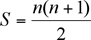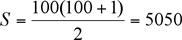# How to add number from 1 to 100 in few seconds math magic EP 17

English version for this video

A famous mathematician, Gauss.  In elementary school in the late 1700’s, Gauss was asked to find the sum of the numbers from 1 to 100.  The question was assigned as “busy work” by the teacher, but Gauss found the answer rather quickly by discovering a pattern.  His observation was as follows:
1 + 2 + 3 + 4 + … + 98 + 99 + 100
Gauss noticed that if he was to split the numbers into two groups (1 to 50 and 51 to 100), he could add them together vertically to get a sum of 101.
1     + 2   + 3   + 4   + 5   + … + 48 + 49 + 50
100 + 99 + 98 + 97 + 96 + … + 53 + 52 + 51
1 + 100 = 101
2 + 99 = 101
3 + 98 = 101
.
.
.
48 + 53 = 101
49 + 52 = 101
50 + 51 = 101
Gauss realized then that his final total would be 50(101) = 5050.
The sequence of numbers (1, 2, 3, … , 100) is arithmetic and when we are looking for the sum of a sequence, we call it a series.  Thanks to Gauss, there is a special formula we can use to find the sum of a series:S is the sum of the series and n is the number of terms in the series, in this case, 100.watch full vedic math videos on- http://youtube.com/rosehubTV

## Unknown

Developer, Educator, Youtuber

I love to spend time with kids, Exploring Internet, Developing Website and Youtbe Videos